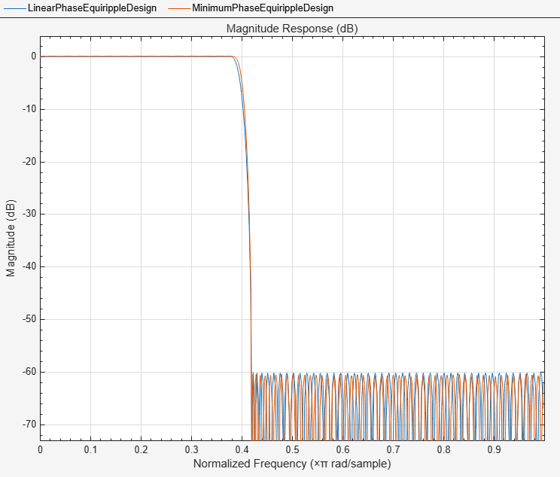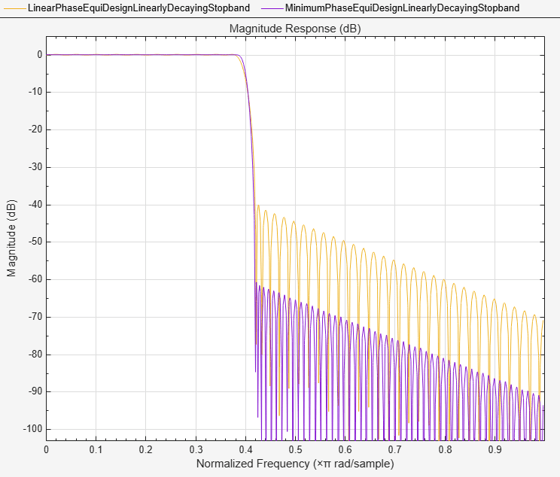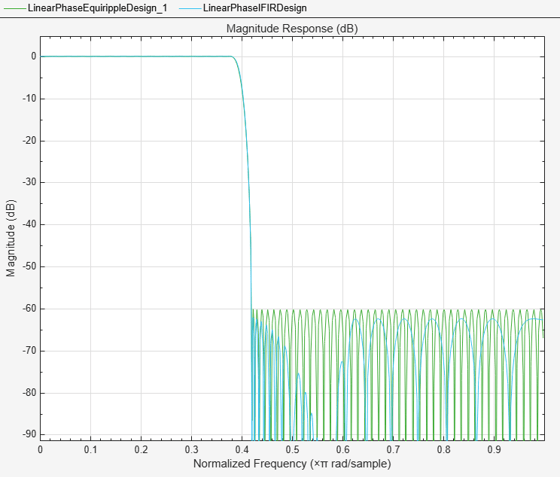## Minimizing Lowpass FIR Filter Length

This example shows how to minimize the number coefficients, by designing minimum-phase or minimum-order filters.

### Minimum-Phase Lowpass Filter Design

To start, set up the filter parameters and use `fdesign` to create a constructor for designing the filter.

``` N = 100; Fp = 0.38; Fst = 0.42; Ap = 0.06; Ast = 60; Hf = fdesign.lowpass('Fp,Fst,Ap,Ast',Fp,Fst,Ap,Ast);```

So far, we have only considered linear-phase designs. Linear phase is desirable in many applications. Nevertheless, if linear phase is not a requirement, minimum-phase designs can provide significant improvements over linear phase counterparts. For instance, returning to the minimum order case, a minimum-phase/minimum-order design for the same specifications can be computed with:

``` Hd1 = design(Hf,'equiripple','systemobject',true); Hd2 = design(Hf,'equiripple','minphase',true,... 'systemobject',true); hfvt = fvtool(Hd1,Hd2,'Color','White'); legend(hfvt,'Linear-phase equiripple design',... 'Minimum-phase equiripple design')```Notice that the number of coefficients has been reduced from 146 to 117. As a second example, consider the design with a stopband decaying in linear fashion. Notice the increased stopband attenuation. The passband ripple is also significantly smaller.

``` setspecs(Hf,'N,Fp,Fst',N,Fp,Fst); Hd3 = design(Hf,'equiripple','StopbandShape','linear',... 'StopbandDecay',53.333,'systemobject',true); setspecs(Hf,'Fp,Fst,Ap,Ast',Fp,Fst,Ap,Ast); Hd4 = design(Hf,'equiripple','StopbandShape','linear',... 'StopbandDecay',53.333,'minphase',true,'systemobject',true); hfvt2 = fvtool(Hd3,Hd4,'Color','White'); legend(hfvt2,'Linear-phase equiripple design with linearly decaying stopband',... 'Minimum-phase equiripple design with linearly decaying stopband')```### Minimum-Order Lowpass Filter Design Using Multistage Techniques

A different approach to minimizing the number of coefficients that does not involve minimum-phase designs is to use multistage techniques. Here we show an interpolated FIR (IFIR) approach.

``` Hd5 = ifir(Hf); hfvt3 = fvtool(Hd1,Hd5,'Color','White'); legend(hfvt3,'Linear-phase equirriple design',... 'Linear-phase IFIR design')```The number of nonzero coefficients required in the IFIR case is 111. Less than both the equiripple linear-phase and minimum-phase designs.

Watch now